# Bellman-Harris process

(diff) ← Older revision | Latest revision (diff) | Newer revision → (diff)

A special case of an age-dependent branching process (cf. Branching process, age-dependent). It was first studied by R. Bellman and T.E. Harris . In the Bellman–Harris process it is assumed that particles live, independently of each other, for random periods of time, and produce a random number of new particles at the end of their life time. If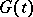is the distribution function of the life times of the individual particles, if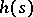is the generating function of the number of direct descendants of one particle, and if at time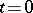the age of the particle was zero, then the generating functionof the number of particlessatisfies the non-linear integral equation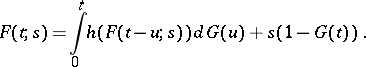If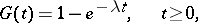the Bellman–Harris process is a Markov branching process with continuous time.

How to Cite This Entry:
Bellman-Harris process. Encyclopedia of Mathematics. URL: http://encyclopediaofmath.org/index.php?title=Bellman-Harris_process&oldid=14293
This article was adapted from an original article by B.A. Sevast'yanov (originator), which appeared in Encyclopedia of Mathematics - ISBN 1402006098. See original article